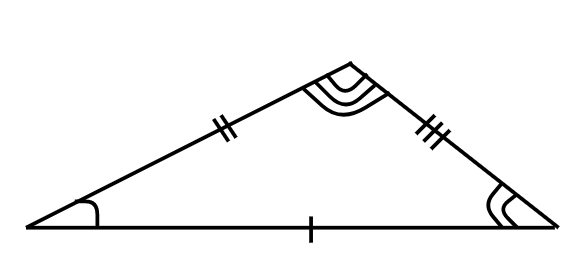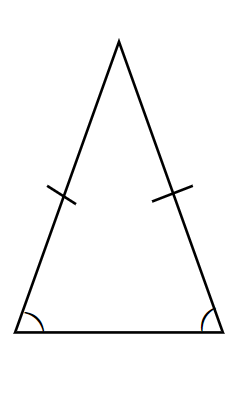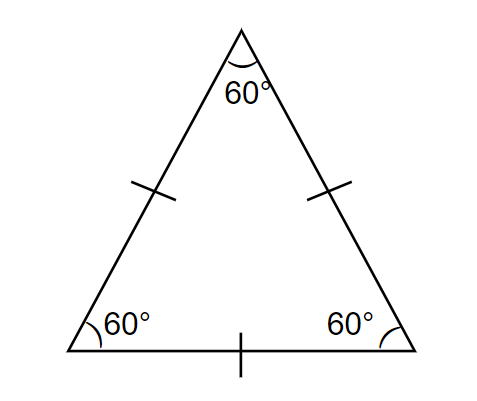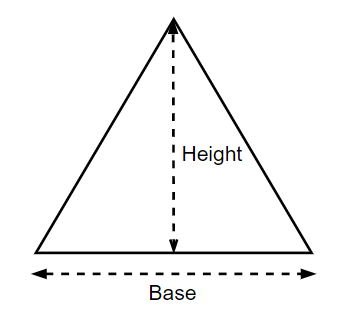QUESTION

# Area of a scalene triangle is given by the formula:A. $\dfrac{1}{2}\times base\times height$ B. $\dfrac{\sqrt{3}}{2}\times {{\left( side \right)}^{2}}$ C. $\dfrac{1}{4}\times base\times \sqrt{4{{\left( side \right)}^{2}}-{{\left( base \right)}^{2}}}$ D. None of the above.

Hint: First, try to recollect the different types of triangles based on the sides and their areas. Now, out of them select the area which is the most appropriate one for the scalene triangle.

In this question, we have to find the formula for the area of a scalene triangle. Before proceeding with the question, let us first know about the different types of triangle based on their sides. According to the length of their sides, triangles can be classified into three types which are: Scalene, Isosceles and Equilateral.
Scalene triangles have all the side lengths of different measures. No side will be equal in length to the other in such a triangle.In an isosceles triangle, the length of two sides are equal.In an equilateral triangle, all the lengths of the sides are equal to each other.Now let us consider the general area of a triangle.The area is half of the base times height. So, area $=\dfrac{1}{2}\times b\times h$ where, $b$ is the distance along the base and $h$ is the height (measured at right angles to the base).
Now, let us consider our question. Here we have to find the area of a scalene triangle. As we have seen that in a scalene triangle, all the side lengths are of different measures, so we cannot write the area of a scalene triangle in terms of one of its particular sides. Also, there is no particular formula for the area of the scalene triangle as all its sides are unequal. So, we write the area of a scalene triangles in the similar way, we write for any general triangle. So, we get, area of scalene triangle $=\dfrac{1}{2}\times b\times h$, where $b$ is the base of the scalene triangle and $h$ is the height of the scalene triangle.
Hence, option A is the correct answer.

Note: In this question, students can directly reject options B and C because they contain sides and no sides of the scalene triangle are equal. Students must note that the formula of area of triangle $=\dfrac{1}{2}\times b\times h$ is valid for every type of triangle, whether it is equilateral, isosceles or scalene. Also, the formulas for equilateral and isosceles triangles are derived from this formula only.